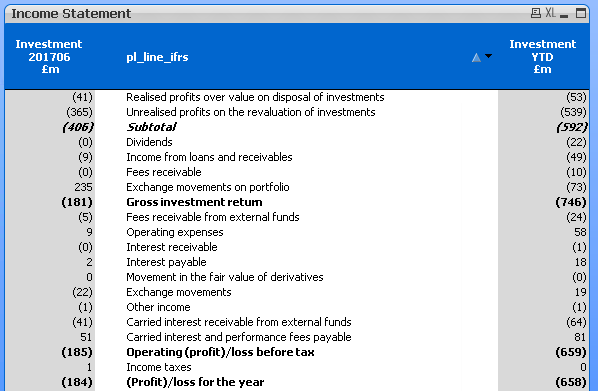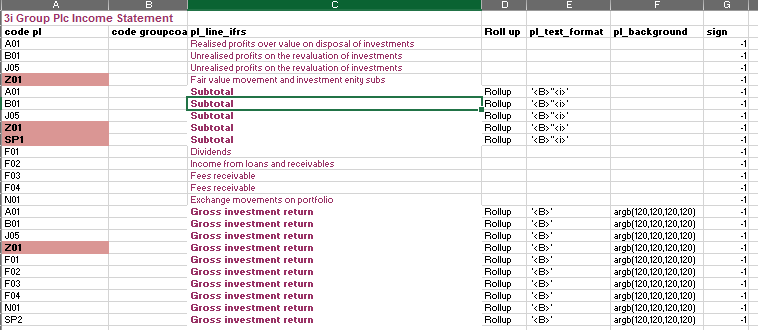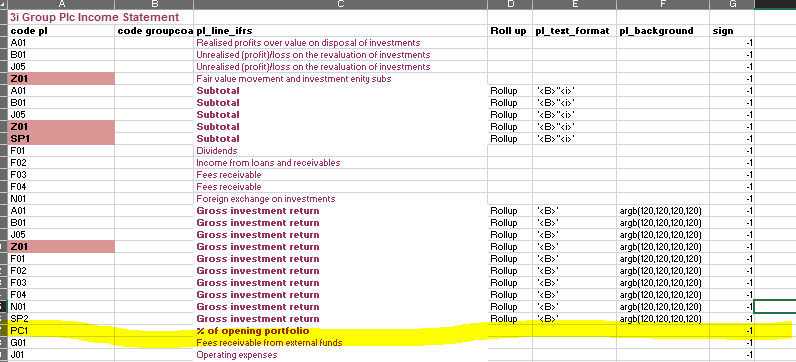# QlikView App Dev

Discussion Board for collaboration related to QlikView App Development.

Announcements
Coming Aug. 9: New Simplified Authoring for Qlik Sense SaaS – For Details, CLICK HERE
cancel
Showing results for
Did you mean:Creator III

## Inserting a calculated %ge line into a straight table

Hello

I have a table below and the layout is based on a table I load from excel.

I would like to add in a line below Gross Investment Return for '% of opening portfolio'

I could add in a line in excel for this, but this line will be a calculation rather than coming from the database.

Do you know how I can add in a row like this?Many thanks

Paul1 Solution

Accepted SolutionsMVP

=If(pl_line_ifrs = '% of opening portfolio',  Sum(TOTAL IS_Investment_GBP), Sum({\$<period = {">=\$(vStartPeriod_CY)<=\$(vEndPeriod_CY)"}>}  \$(vIS_Investment) )/1000000)

4 RepliesMVPCreator III
Author

Hi Sunny

That did help.  I amdended my excel sheet and put in a line '% of opening portfolio'And I amended the YTD column to say

=if( pl_line_ifrs = '% of opening portfolio',  '1',  sum({\$<period = {">=\$(vStartPeriod_CY)<=\$(vEndPeriod_CY)"}>}  \$(vIS_Investment) )/1000000)

Just to test and it did bring back a '1'.

But as soon as I put any simple calculation in here it then doesn't bring back this line eg

=if( pl_line_ifrs = '% of opening portfolio',  sum(IS_Investment_GBP) sum({\$<period = {">=\$(vStartPeriod_CY)<=\$(vEndPeriod_CY)"}>}  \$(vIS_Investment) )/1000000)Do you have any idea why this might be?MVP